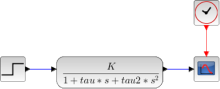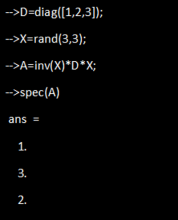# Mathematics

Scilab provides about 1,700 mathematical functions for usual engineering and science applications including mathematical operations and data analysis.

## Elementary functions

```// x initialisation
x=[0:0.1:2*%pi]';
//simple plot
plot(sin(x))```## Polynomials and rational functions

```//seed for defining polynomials
with symbol "s"
s=poly(0,"s");p=1+s+2*s^2;```## Vectors and Matrixes

The core of Scilab is based on linear algebra librairies.
It is optimized for matrix computations.

--> A=[1,2,3]
A  =

1. 2.    3.

–> B=A’*A
B  =

1. 2.    3.
2. 4.    6.
3. 6.    9.